# Reflection principle

A generalization of the symmetry principle for harmonic functions to harmonic functions in an arbitrary number of independent variables. Some formulations of the reflection principle are as follows:

1) Letbe a domain in a-dimensional Euclidean spacethat is bounded by a Jordan surface(in particular, a smooth or piecewise-smooth surfacewithout self-intersections) containing a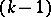-dimensional subdomainof a-dimensional hyperplane. If the functionis harmonic in, continuous on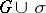and equal to zero everywhere on, then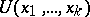can be extended as a harmonic function acrossinto the domainthat is symmetric torelative to, by means of the equalitywhere the points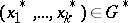and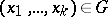are symmetric relative to.

2) Letbe a domain of a-dimensional Euclidean space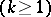that is bounded by a Jordan surfacecontaining a-dimensional subdomainof a-dimensional sphere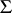of radiuswith centre at a point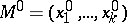. Ifis harmonic in, continuous onand equal to zero everywhere on, thencan be extended as a harmonic function acrossinto the domain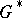that is symmetric torelative to(i.e. obtained fromby means of the transformation of inverse radii — inversions — relative to). This continuation is achieved by means of the Kelvin transformation, taken with the opposite sign, ofrelative to, namely: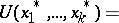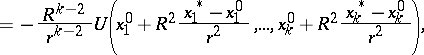where,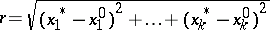. Under the transformation of inverse radii relative to, the point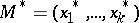is mapped to the point, in correspondence with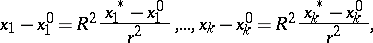such that if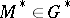, thenbelongs to the domain(whereis given), and if, then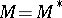.